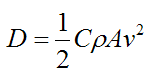# Drag Force

A drag force is the resistance force caused by the motion of a body through a fluid, such as water or air. A drag force acts opposite to the direction of the oncoming flow velocity. This is the relative velocity between the body and the fluid.

The drag force D exerted on a body traveling though a fluid is given byWhere:

C is the drag coefficient, which can vary along with the speed of the body. But typical values range from 0.4 to 1.0 for different fluids (such as air and water)

ρ is the density of the fluid through which the body is moving

v is the speed of the body relative to the fluid

A is the projected cross-sectional area of the body perpendicular to the flow direction (that is, perpendicular to v). This is illustrated in the figure belowTo see an example of a problem that involves drag, go to The physics of skydiving.